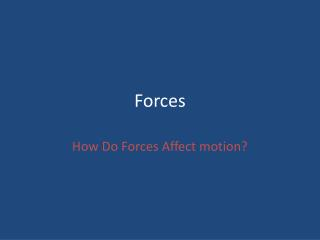DownloadDownload PresentationForces

# Forces

Télécharger la présentation## Forces

- - - - - - - - - - - - - - - - - - - - - - - - - - - E N D - - - - - - - - - - - - - - - - - - - - - - - - - - -
##### Presentation Transcript

1. Forces How Do Forces Affect motion?

2. Force • A push or a pull • Force can cause an object to change speed or direction • A Force is measured in Newtons • 1 N =1 kg x 1 m/s2 • Force = Mass x acceleration • Newton’s 2nd law

3. Net Force • Combination of all forces acting on the object • Forces acting in the same direction: add together

4. Unbalanced Force • Unbalanced force causes a change in the objects motion. Net force is NOT 0 Newtons

5. Balanced Force • Net force is 0 Newtons

6. Net force = 90 N

7. Newton’s Second law • The acceleration of an object depends on the mass of an object and the amount of force applied. • F=ma • F= force; m= mass (kg); a= acceleration (km/s2) • What is the force? 24 kg x 40 m/s2 =N

8. Newton’s 1st law • Law of inertia • An object at rest stays at rest, an object in motion stays in motion at the same speed unless, acted upon by an unbalanced force.

9. Why is motorcyclist flying over tires?

10. Newton’s third law • Whenever an object exerts a force on a second object, the second object exerts an equal and opposite force on the first.

11. Newton’s third law

12. Newton’s 3rd law • Forces in pairs have equal size but opposite directions